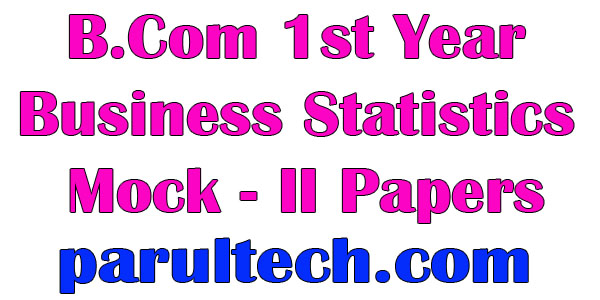# B.Com 1st Year Business Statistics Mock – II Papers

B.Com 1st Year Business Statistics Mock II Papers :- Notes Study material Unit Wise Chapter Wise Topic Wise division of the content. Question Answer Study Material Notes Examination Paper Sample Model Paper Previous Year Paper PDF Download This Post is very useful for all the Student B.A., B.Sc., B.Com., M.A., M.Com.### Section-A

Note: This Section contains one question of ten parts requiring short answers. Each part carries 4 marks. (4×10=40 1 .
1. (i) What are the main functions of C.S.O.?
(ii) What is Fisher’s ideal index number? Why is it called ideal? (iii) In a batch of 15 students, 5 students failed in a test. The marks of 10 students who passed were 9,6,7,8,8,9,6,5,4, 7. What was the median of the marks of all the 15 students? (iv) In a frequency distribution, the coefficient of skewness based on quartiles is 0.6. If the sum o: the upper and lower quartiles is 100 and median is 38, find the value of both quartiles. (v) Define probable error. Describe the interpretation of coefficient of correlation. (vi) What do you mean by splicing? Explain. (vii) What is a time series? Explain the objectives of the analysis of a time series. (viii) If the coefficient of correlation of two variables is 0.9 and number of pairs of pairs of items is 18, calculate the probable error. (ix) What are the considerations that influence the selection of an appropriate average: (x) How can you locate mode by graphic method? : ”

Section B, C, D & E

Note: Each section contains two questions. Attempt one question from each section. Each question came 15 marks. Answer must be descriptive. (15×4=60)

Section B

1. A train starts from a place and covers quarters of a km at the speed of 12, 16, 24 and 48 km per hour respectively. Average speed for the journey of km is 19.2 km per hour and not 25 km per hour. Explain this fact in a manner as you will tell to a layman and verify the correctness of calculation.
2. What is correlation? How would you use the following methods in determining correlation?
(i) Karl Pearson’s coefficient of correlation. (ii) Spearman’s rank difference method. (iii) Coefficient of concurrent deviation method.

Section C

1. Statistics is not science, it is a scientific method. Discuss briefly explaining the scope, utility and limitations of statistics.
2. Find out both quartiles, fourth decile and seventy fourth percentile from the following data:

 Marks 30 40 50 60 70 80 90 No. Of students 4 6 11 19 20 26 13

Section D

1. Find range and coefficient of range from the distribution:

 Class 6-10 11-15 16-20 21-25 26-30 31-35 36-40 Frequency 7 8 15 35 18 7 5

1. What is time series? Explain the various components of time series. Also discuss its importance.

Section E

1. Elaborate on the tests that help to determine the adequacy of an index number formula.
2. Explain the importance of graphic presentation of data. Describe the rules of graphic presentation and also explain its advantages and disadvantages.